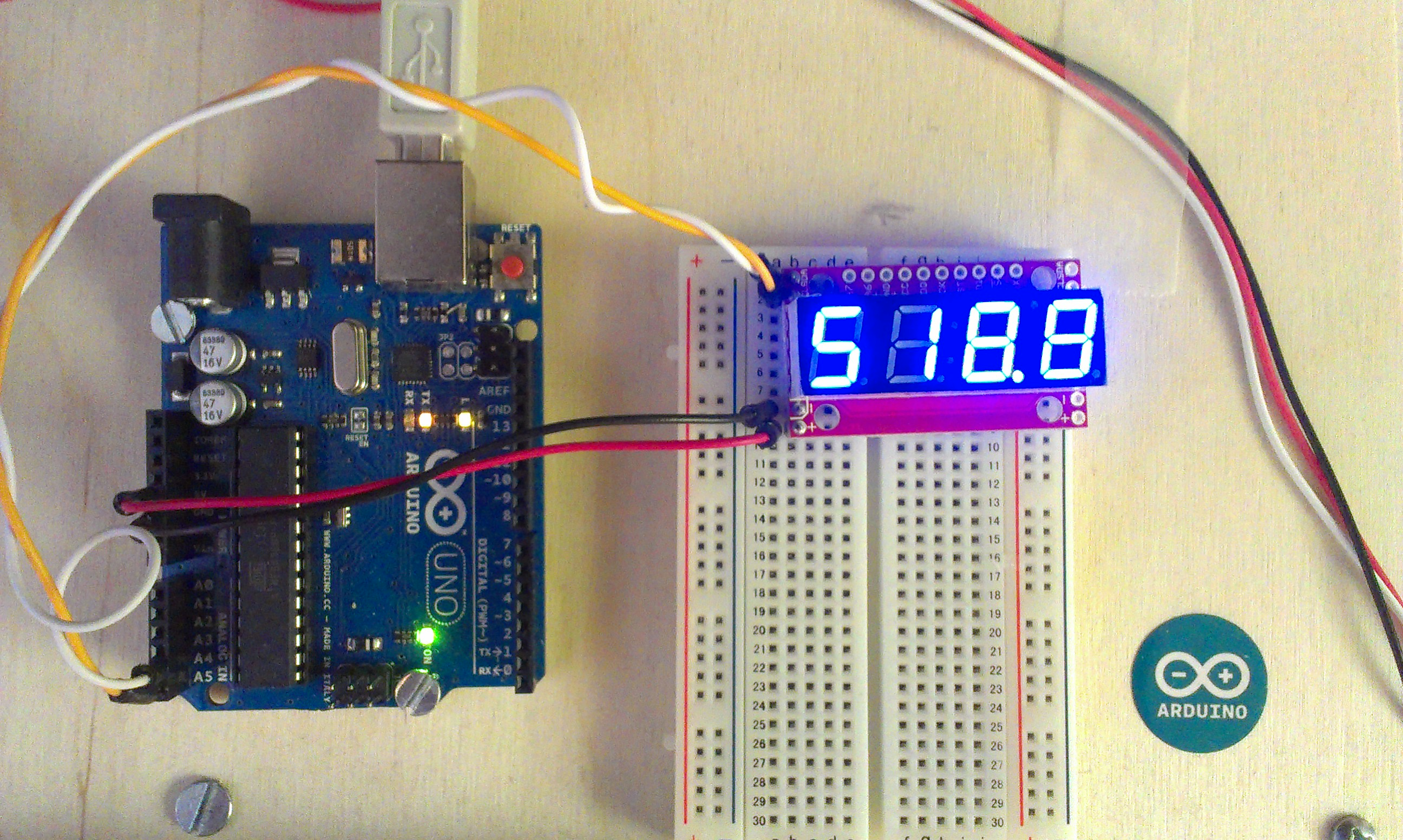# Clock Display With Serial - Sparkfun

Clock Display With Serial - Sparkfun

### Introduction

These seven segment displays are managed by an extra Arduino embedded in them. As a result, far less wiring and code is required for your project, since it is all encapsulated in this nice package. Here is the finished project:### Basic Wiring (I2C)

Connect These Pins:
ArduinoSerial7Segment
A5 SCL
A4 SDA
5V +
GND -

The wiring is really straightforward. On the top sides there is an SDA and SCL, which go to A4 and A5 respectively on your Arduino Uno. If you are using a different Arduino, check the documentation on the Wire library to see which pin you should use.

On the bottom sides, there is a plus and minus, which should be hooked up to the 5V and GND pins respectively on your Arduino.

### Basic Code

I based my code on the public domain code posted by sparkfun. I added:

• Number formatting (space padded to the right)
• Funciton for decimal printing using floats
• Beware! Floats on Arduino are really innacurate. I get 0.01 error on just a 3 digit number!
```/* Serial7Segment is an open source seven segment display.
To get this code to work, attach a Serial7Segment to an Arduino Uno using:
A5 to SCL
A4 to SDA
VIN to PWR
GND to GND
*/

#include <Wire.h>

#define APOSTROPHE  5
#define COLON       4
#define DECIMAL4    3
#define DECIMAL3    2
#define DECIMAL2    1
#define DECIMAL1    0

//This is the default address of the OpenSegment with both solder jumpers open

void setup()  {
Wire.begin();       //Join the bus as master

Serial.begin(9600); //Start USB serial communication at 9600 for debug prints
//Send the reset command to the display  //this forces the cursor to return to the beginning of the display
Wire.write('v');
Wire.endTransmission();

//High Brightness
Wire.write(0x7A);         // Brightness control command
Wire.write(100);          // Set brightness level: 0% to 100%
Wire.endTransmission();
}

float cycles = 0.0;         // TEST DECIMAL SENDING
void loop() {
cycles+= 0.1;             // increment decimal
Serial.print("Cycle: ");
Serial.println(cycles);

i2cSend(cycles);          //Send the decimal to the display

delay(1);                 //a very small delay to prevent flickering
}
void i2cSend(float numberToPrint) {
int decimalPlace = -1;
//find decimal place, fix the position of leftmost digit to the left
if ( numberToPrint < 0 ) {
i2cSend( int( numberToPrint * 100 ) );
decimalPlace = DECIMAL1;
}
else if ( numberToPrint < 10 ) {
i2cSend( int( numberToPrint * 1000 ) );
decimalPlace = DECIMAL1;
}
else if ( numberToPrint < 100 ) {
i2cSend( int( numberToPrint * 100) );
decimalPlace = DECIMAL2;
}
else if ( numberToPrint < 1000 ) {
i2cSend( int( numberToPrint * 10) );
decimalPlace = DECIMAL3;
}
else {
i2cSend( numberToPrint );
}

if ( decimalPlace != -1 ) {
Wire.beginTransmission(DISPLAY_ADDRESS1); // transmit to device #1
Wire.write( 0x77 );                       // send decimal command
Wire.write( 1 << decimalPlace );          // send the place using bitshift
Wire.endTransmission();                   // Stop I2C transmission
}
}

//Given a number, chop up an integer into four values and sends them over I2C
void i2cSend(int numberToPrint ) {  // use snprintf. It will align the number to the right of the string  // snprintf truncates the number after the first few digits  char str;
snprintf(str, 5, "%4d", numberToPrint);    i2cSendString( str );  // the number is too big - mark that it was cut off.
if ( numberToPrint > 9999 ) {      Wire.beginTransmission(DISPLAY_ADDRESS1);   // transmit to device #1      Wire.write( 0x77 );                         // send decimal command
Wire.write( 1 << APOSTROPHE );              // indicate an "overflow"      Wire.endTransmission();                     // Stop I2C transmission
}
}

//Given a string, i2cSendString sends the first four characters over i2c
void i2cSendString(char *toSend) {
Wire.beginTransmission(DISPLAY_ADDRESS1); // transmit to device #1
for(byte x = 0 ; x < 4 ; x++)             // for each of the 4 characters
Wire.write(toSend[x]);                  // Send the character from the array
Wire.endTransmission();                   // Stop I2C transmission
}```

### Documentation

Here are some other links to SparkFun's documentation:

Evan Boldt Thu, 02/14/2013 - 09:46